# Chapter 7 Network Flow Slides by Kevin Wayne

• Slides: 50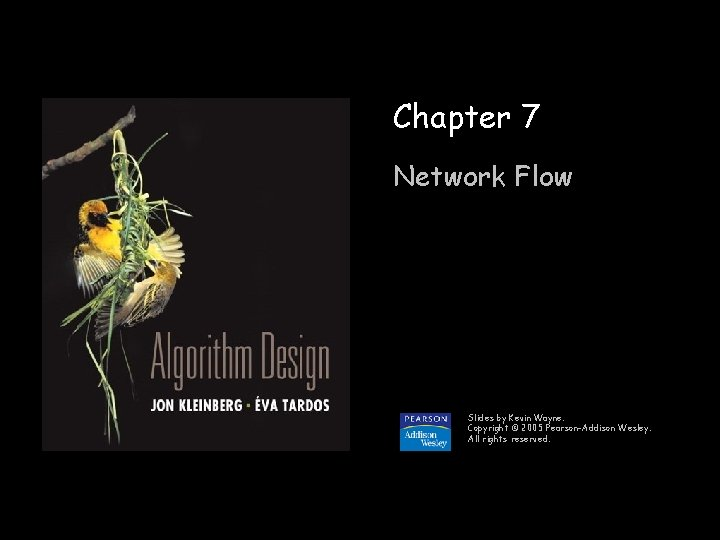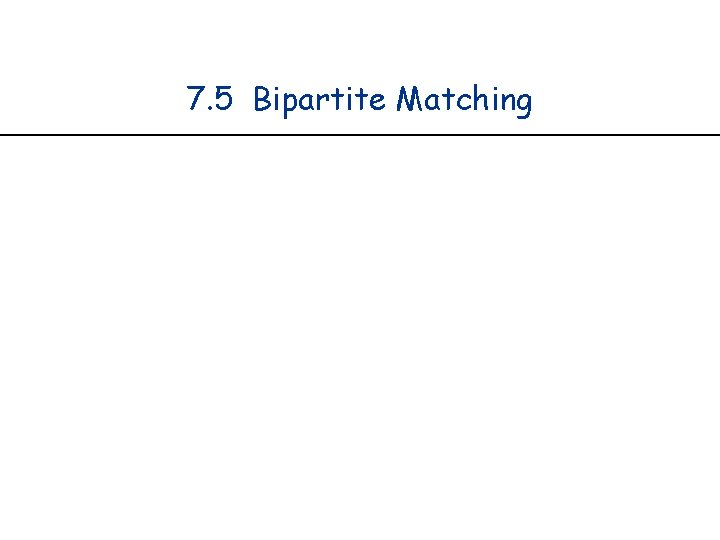7. 5 Bipartite Matching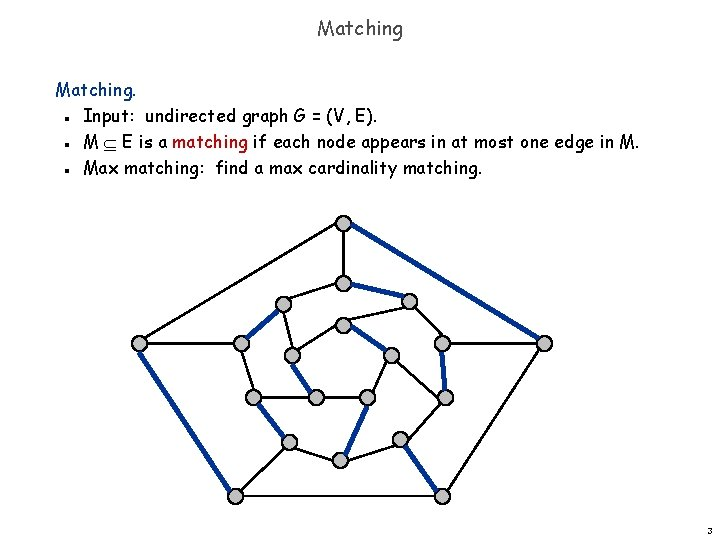Matching. Input: undirected graph G = (V, E). M E is a matching if each node appears in at most one edge in M. Max matching: find a max cardinality matching. n n n 3Bipartite Matching Bipartite matching. Input: undirected, bipartite graph G = (L R, E). n – bipartite graph (or bigraph) is a graph whose vertices can be divided into two disjoint sets (U and V) such that every edge connects a vertex in U to one in V. n n M E is a matching if each node appears in at most one edge in M. Max matching: find a max cardinality matching. 1 1' matching L 2 2' 3 3' 4 4' 5 5' 1 -2', 3 -1', 4 -5' R 4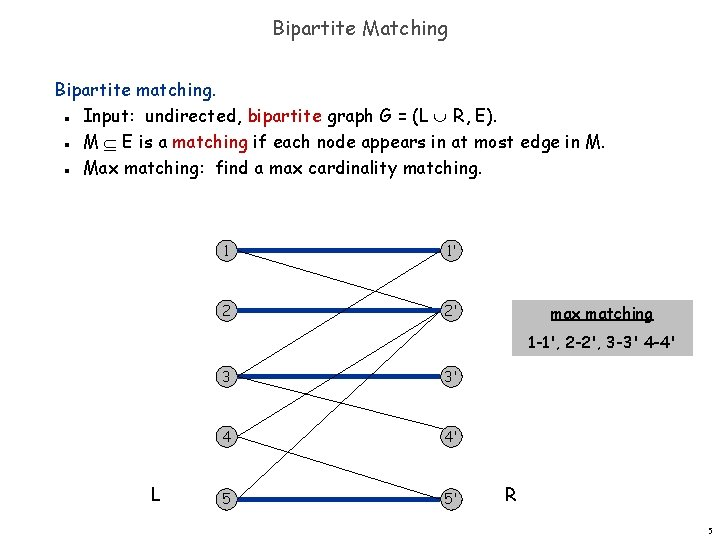Bipartite Matching Bipartite matching. Input: undirected, bipartite graph G = (L R, E). M E is a matching if each node appears in at most edge in M. Max matching: find a max cardinality matching. n n n 1 1' 2 2' max matching 1 -1', 2 -2', 3 -3' 4 -4' L 3 3' 4 4' 5 5' R 5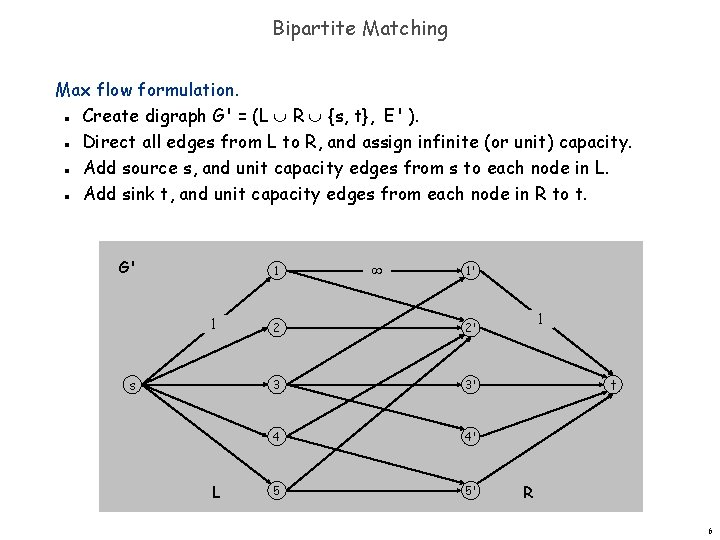Bipartite Matching Max flow formulation. Create digraph G' = (L R {s, t}, E' ). Direct all edges from L to R, and assign infinite (or unit) capacity. Add source s, and unit capacity edges from s to each node in L. Add sink t, and unit capacity edges from each node in R to t. n n G' 1 1 s L 1' 2 2' 3 3' 4 4' 5 5' 1 t R 6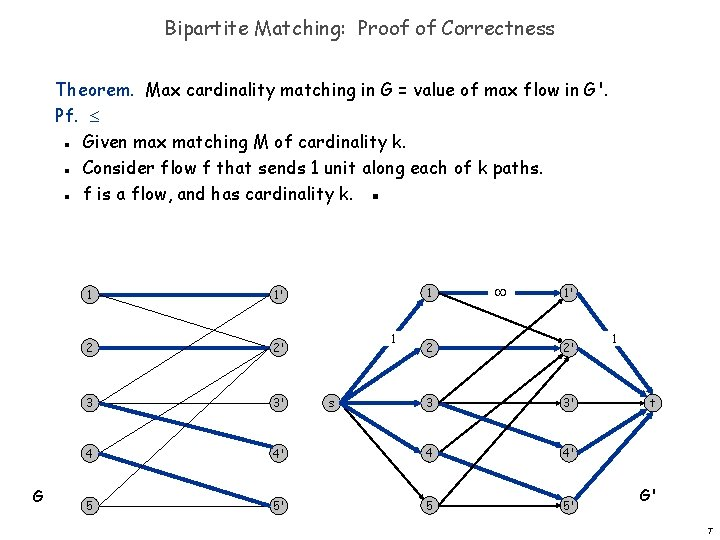Bipartite Matching: Proof of Correctness Theorem. Max cardinality matching in G = value of max flow in G'. Pf. Given max matching M of cardinality k. Consider flow f that sends 1 unit along each of k paths. f is a flow, and has cardinality k. ▪ n n n G 1 1' 2 2' 3 3' 4 5 1 1 1' 2 2' 3 3' 4' 4 4' 5' 5 5' s 1 t G' 7Bipartite Matching: Proof of Correctness Theorem. Max cardinality matching in G = value of max flow in G'. Pf. Let f be a max flow in G' of value k. Integrality theorem k is integral and can assume f is 0 -1. Consider M = set of edges from L to R with f(e) = 1. – each node in L and R participates in at most one edge in M – |M| = k: consider cut (L s, R t) ▪ n n n 1 1 s G' 1' 1 1 1' 2 2' 3 3' 4 4' 5 5' t G 8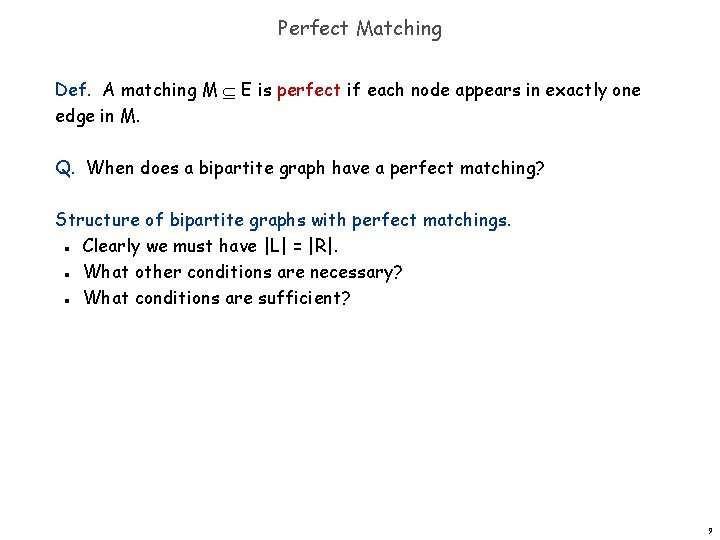Perfect Matching Def. A matching M E is perfect if each node appears in exactly one edge in M. Q. When does a bipartite graph have a perfect matching? Structure of bipartite graphs with perfect matchings. Clearly we must have |L| = |R|. What other conditions are necessary? What conditions are sufficient? n n n 9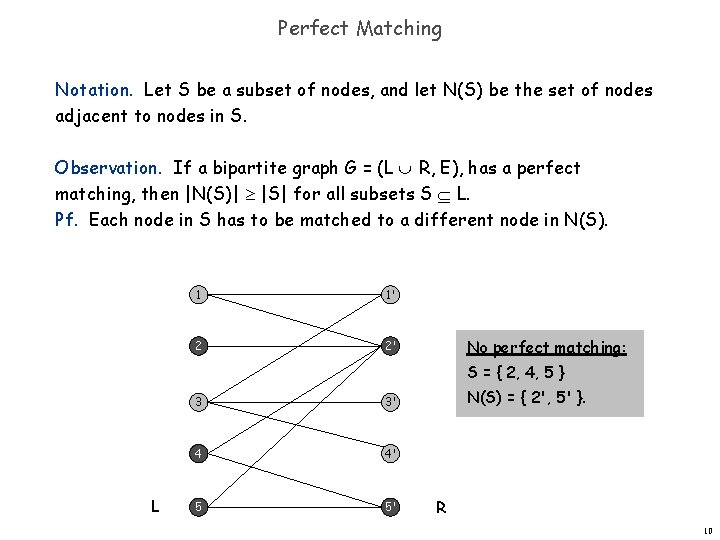Perfect Matching Notation. Let S be a subset of nodes, and let N(S) be the set of nodes adjacent to nodes in S. Observation. If a bipartite graph G = (L R, E), has a perfect matching, then |N(S)| |S| for all subsets S L. Pf. Each node in S has to be matched to a different node in N(S). 1 1' 2 2' No perfect matching: S = { 2, 4, 5 } L 3 3' 4 4' 5 5' N(S) = { 2', 5' }. R 10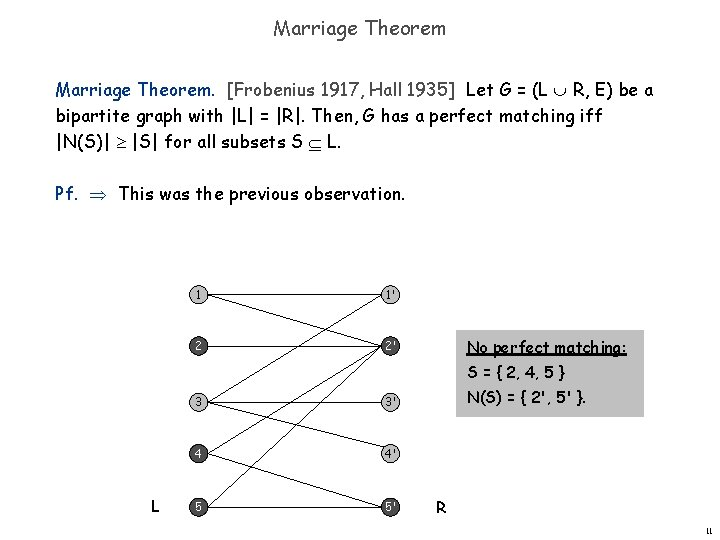Marriage Theorem. [Frobenius 1917, Hall 1935] Let G = (L R, E) be a bipartite graph with |L| = |R|. Then, G has a perfect matching iff |N(S)| |S| for all subsets S L. Pf. This was the previous observation. 1 1' 2 2' No perfect matching: S = { 2, 4, 5 } L 3 3' 4 4' 5 5' N(S) = { 2', 5' }. R 11Proof of Marriage Theorem Pf. Suppose G does not have a perfect matching. Formulate as a max flow problem and let (A, B) be min cut in G'. By max-flow min-cut, cap(A, B) < | L |. Define LA = L A, LB = L B , RA = R A. cap(A, B) = | LB | + | RA |. Since min cut can't use edges: N(LA) RA. |N(LA )| | RA | = cap(A, B) - | LB | < | L | - | LB | = | LA |. – Choose S = LA , conflict with assumption ▪ n n n 1 G' 1 1' A 2 2' LA = {2, 4, 5} 1 LB = {1, 3} s 1 4 5 4' 5' RA = {2', 5'} 3' 3 t N(LA) = {2', 5'} 1 12Bipartite Matching: Running Time Which max flow algorithm to use for bipartite matching? Generic augmenting path: O(m val(f*) ) = O(mn). Capacity scaling: O(m 2 log C ) = O(m 2). Shortest augmenting path: O(m n 1/2). n n n Non-bipartite matching. Structure of non-bipartite graphs is more complicated, but well-understood. [Tutte-Berge, Edmonds-Galai] Blossom algorithm: O(n 4). [Edmonds 1965] Best known: O(m n 1/2). [Micali-Vazirani 1980] n n n 13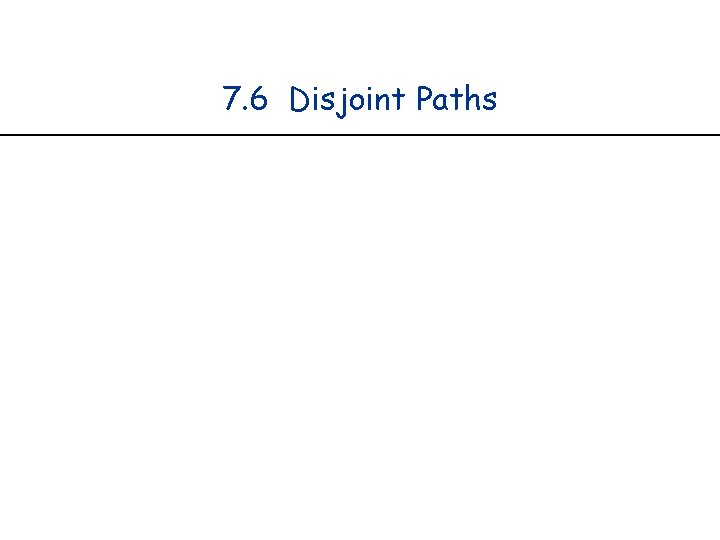7. 6 Disjoint Paths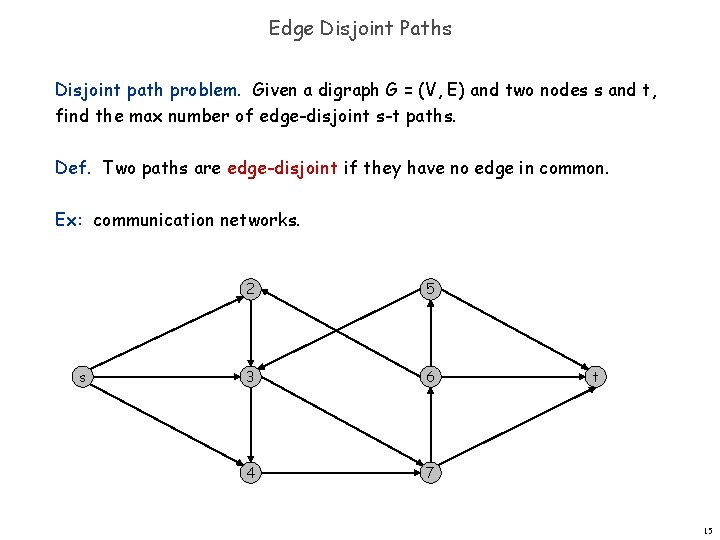Edge Disjoint Paths Disjoint path problem. Given a digraph G = (V, E) and two nodes s and t, find the max number of edge-disjoint s-t paths. Def. Two paths are edge-disjoint if they have no edge in common. Ex: communication networks. s 2 5 3 6 4 7 t 15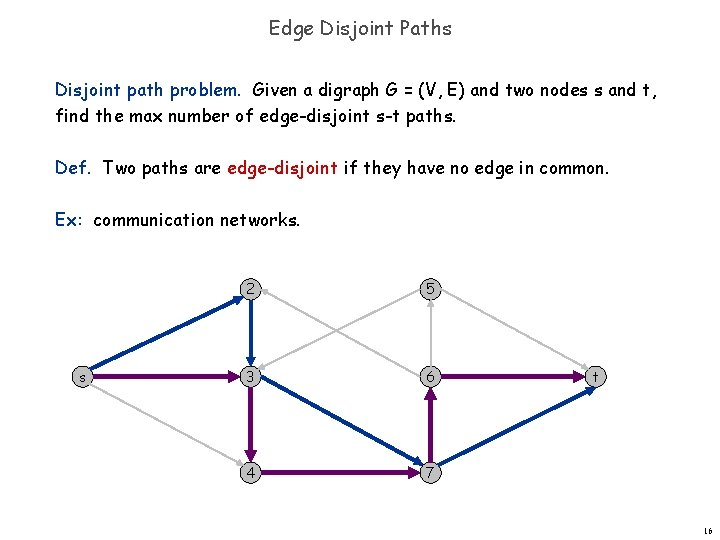Edge Disjoint Paths Disjoint path problem. Given a digraph G = (V, E) and two nodes s and t, find the max number of edge-disjoint s-t paths. Def. Two paths are edge-disjoint if they have no edge in common. Ex: communication networks. s 2 5 3 6 4 7 t 16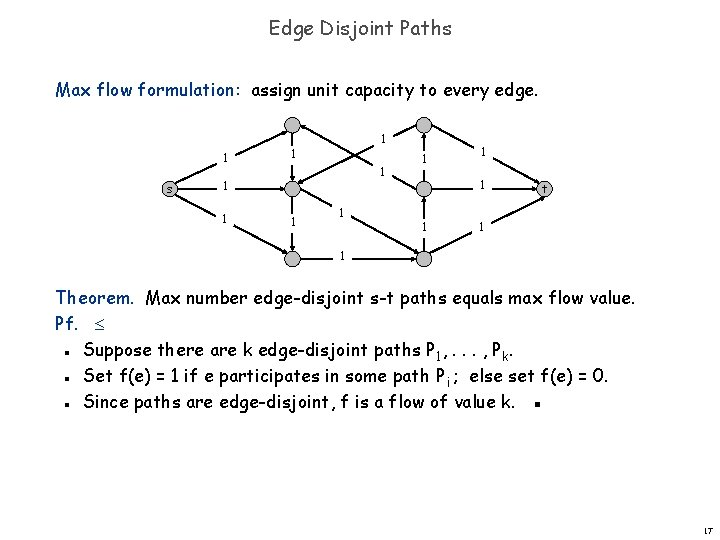Edge Disjoint Paths Max flow formulation: assign unit capacity to every edge. 1 s 1 1 1 t 1 1 Theorem. Max number edge-disjoint s-t paths equals max flow value. Pf. Suppose there are k edge-disjoint paths P 1, . . . , Pk. Set f(e) = 1 if e participates in some path Pi ; else set f(e) = 0. Since paths are edge-disjoint, f is a flow of value k. ▪ n n n 17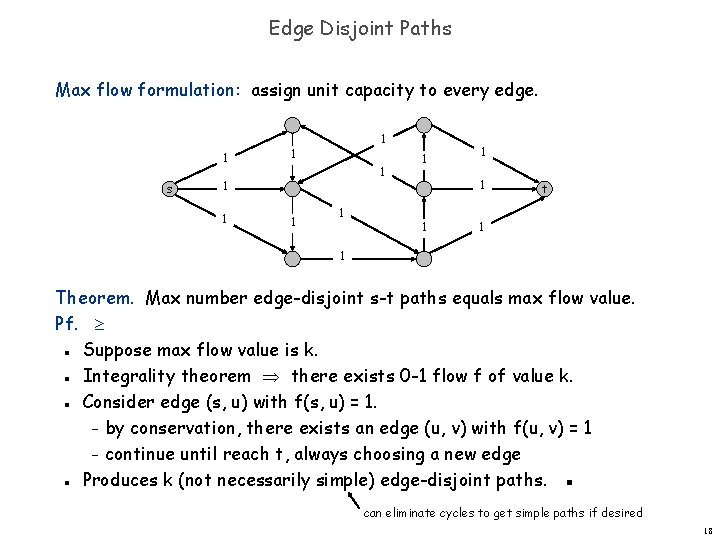Edge Disjoint Paths Max flow formulation: assign unit capacity to every edge. 1 s 1 1 1 t 1 1 Theorem. Max number edge-disjoint s-t paths equals max flow value. Pf. Suppose max flow value is k. Integrality theorem there exists 0 -1 flow f of value k. Consider edge (s, u) with f(s, u) = 1. – by conservation, there exists an edge (u, v) with f(u, v) = 1 – continue until reach t, always choosing a new edge Produces k (not necessarily simple) edge-disjoint paths. ▪ n n can eliminate cycles to get simple paths if desired 18Network Connectivity Network connectivity. Given a digraph G = (V, E) and two nodes s and t, find min number of edges whose removal disconnects t from s. Def. A set of edges F E disconnects t from s if every s-t path uses at least one edge in F. s 2 5 3 6 4 7 t 19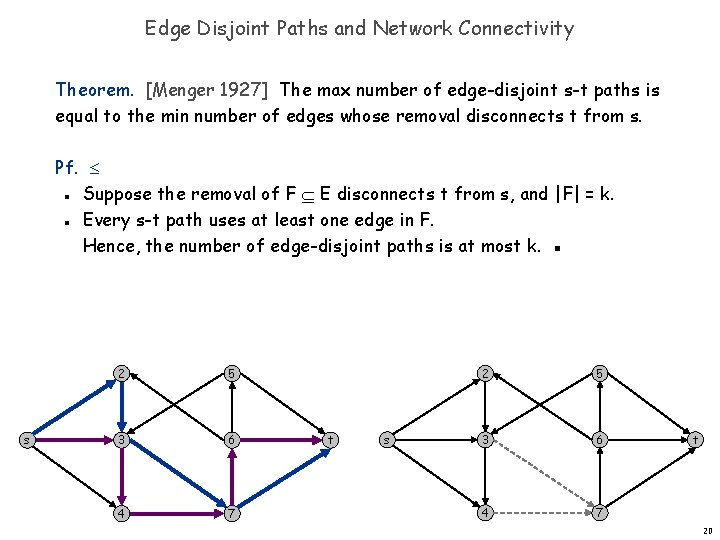Edge Disjoint Paths and Network Connectivity Theorem. [Menger 1927] The max number of edge-disjoint s-t paths is equal to the min number of edges whose removal disconnects t from s. Pf. Suppose the removal of F E disconnects t from s, and |F| = k. Every s-t path uses at least one edge in F. Hence, the number of edge-disjoint paths is at most k. ▪ n n s 2 5 3 6 4 7 t 20Disjoint Paths and Network Connectivity Theorem. [Menger 1927] The max number of edge-disjoint s-t paths is equal to the min number of edges whose removal disconnects t from s. Pf. Suppose max number of edge-disjoint paths is k. Then max flow value is k. Max-flow min-cut (A, B) of capacity k. Let F be set of edges going from A to B. |F| = k and disconnects t from s. ▪ n n n A s 2 5 3 6 4 7 t 217. 7 Extensions to Max Flow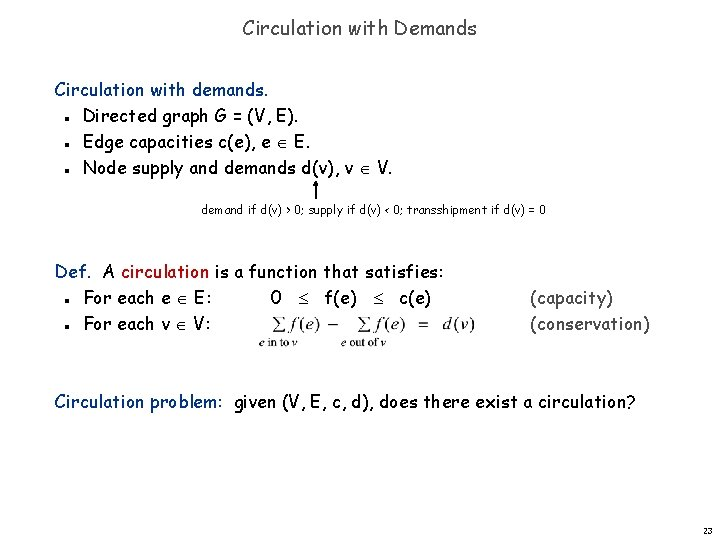Circulation with Demands Circulation with demands. Directed graph G = (V, E). Edge capacities c(e), e E. Node supply and demands d(v), v V. n n n demand if d(v) > 0; supply if d(v) < 0; transshipment if d(v) = 0 Def. A circulation is a function that satisfies: For each e E: 0 f(e) c(e) For each v V: n n (capacity) (conservation) Circulation problem: given (V, E, c, d), does there exist a circulation? 23Circulation with Demands Necessary condition: sum of supplies = sum of demands. Pf. Sum conservation constraints for every demand node v. -6 -8 6 7 4 10 -7 6 6 1 7 7 9 4 2 3 3 10 supply 0 11 4 4 capacity demand flow 24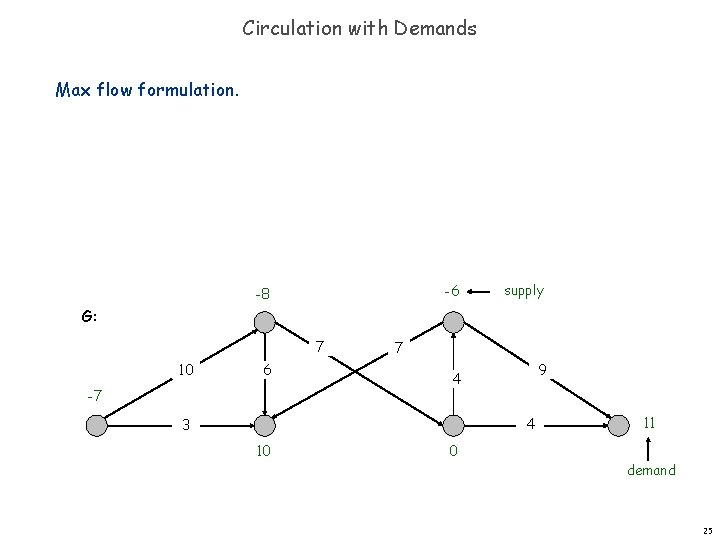Circulation with Demands Max flow formulation. -6 -8 supply G: 7 10 6 -7 7 9 4 4 3 10 0 11 demand 25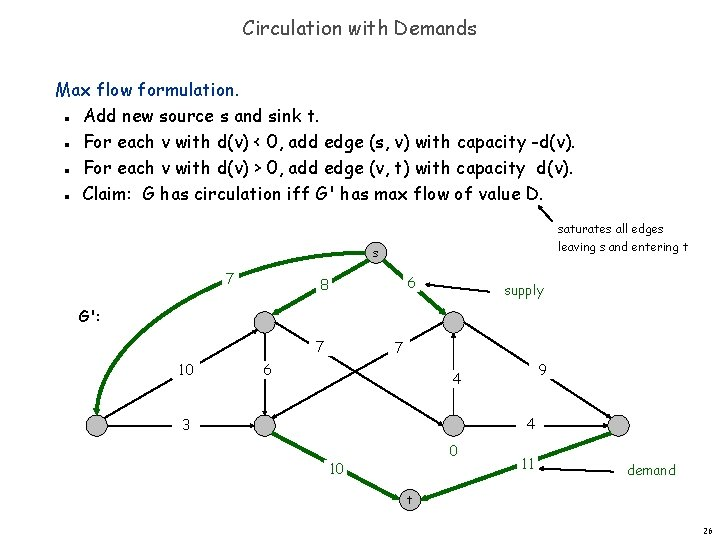Circulation with Demands Max flow formulation. Add new source s and sink t. For each v with d(v) < 0, add edge (s, v) with capacity -d(v). For each v with d(v) > 0, add edge (v, t) with capacity d(v). Claim: G has circulation iff G' has max flow of value D. n n saturates all edges leaving s and entering t s 7 6 8 supply G': 7 10 7 6 9 4 4 3 0 10 11 demand t 26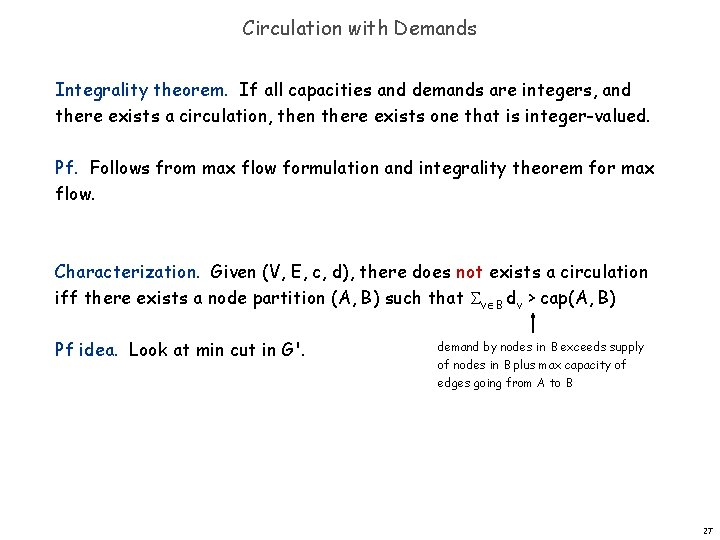Circulation with Demands Integrality theorem. If all capacities and demands are integers, and there exists a circulation, then there exists one that is integer-valued. Pf. Follows from max flow formulation and integrality theorem for max flow. Characterization. Given (V, E, c, d), there does not exists a circulation iff there exists a node partition (A, B) such that v B dv > cap(A, B) Pf idea. Look at min cut in G'. demand by nodes in B exceeds supply of nodes in B plus max capacity of edges going from A to B 27Circulation with Demands and Lower Bounds Feasible circulation. Directed graph G = (V, E). Edge capacities c(e) and lower bounds �(e), e E. Node supply and demands d(v), v V. n n n Def. A circulation is a function that satisfies: For each e E: �(e) f(e) c(e) For each v V: n n (capacity) (conservation) Circulation problem with lower bounds. Given (V, E, � , c, d), does there exists a a circulation? 28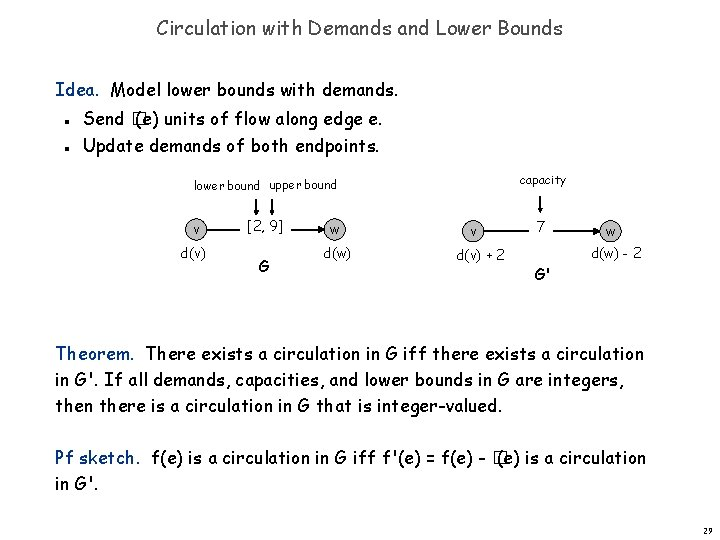Circulation with Demands and Lower Bounds Idea. Model lower bounds with demands. n n Send � (e) units of flow along edge e. Update demands of both endpoints. capacity lower bound upper bound v d(v) [2, 9] G w d(w) v d(v) + 2 7 G' w d(w) - 2 Theorem. There exists a circulation in G iff there exists a circulation in G'. If all demands, capacities, and lower bounds in G are integers, then there is a circulation in G that is integer-valued. Pf sketch. f(e) is a circulation in G iff f'(e) = f(e) - � (e) is a circulation in G'. 29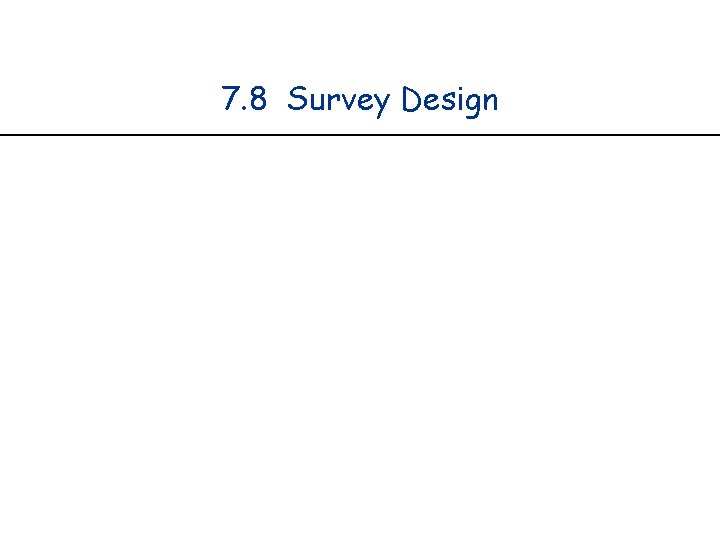7. 8 Survey DesignSurvey Design one survey question per product Survey design. Design survey asking n 1 consumers about n 2 products. Can only survey consumer i about product j if they own it. Ask consumer i between ci and ci' questions. Ask between pj and pj' consumers about product j. n n Goal. Design a survey that meets these specs, if possible. Bipartite perfect matching. Special case when ci = ci' = pi' = 1. 31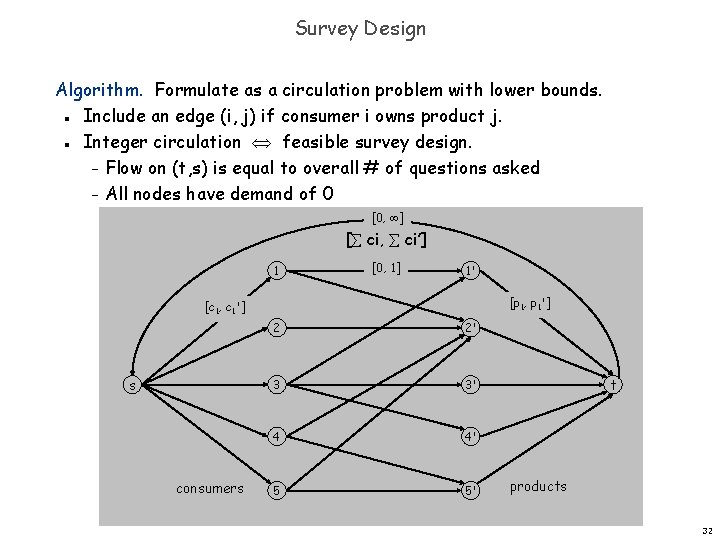Survey Design Algorithm. Formulate as a circulation problem with lower bounds. Include an edge (i, j) if consumer i owns product j. Integer circulation feasible survey design. – Flow on (t, s) is equal to overall # of questions asked – All nodes have demand of 0 n n [0, ] [ ci, ci’] 1 [0, 1] 1' [p 1, p 1'] [c 1, c 1'] s consumers 2 2' 3 3' 4 4' 5 5' t products 32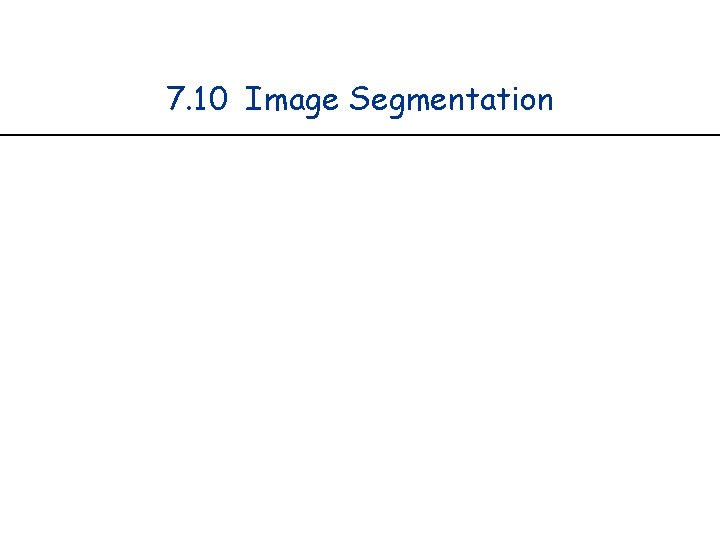7. 10 Image Segmentation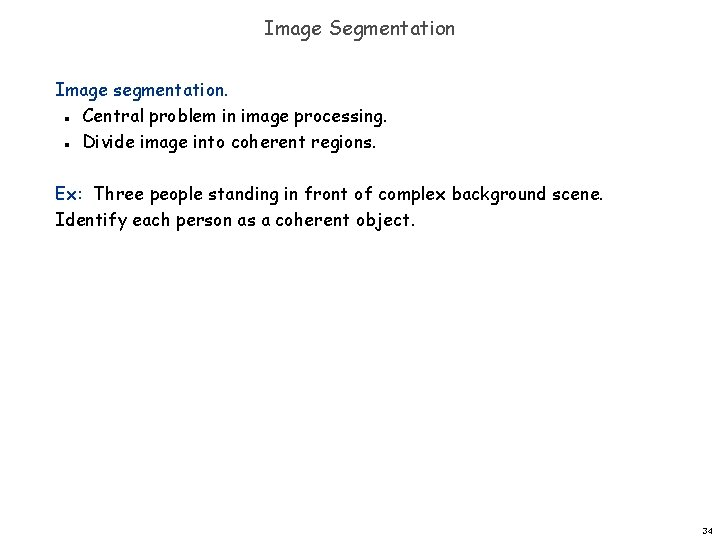Image Segmentation Image segmentation. Central problem in image processing. Divide image into coherent regions. n n Ex: Three people standing in front of complex background scene. Identify each person as a coherent object. 34Image Segmentation Foreground / background segmentation. Label each pixel in picture as belonging to foreground or background. V = set of pixels, E = pairs of neighboring pixels. ai 0 is likelihood pixel i in foreground. bi 0 is likelihood pixel i in background. pij 0 is separation penalty for labeling one of i and j as foreground, and the other as background. n n n Goals. Accuracy: if ai > bi in isolation, prefer to label i in foreground. Smoothness: if many neighbors of i are labeled foreground, we should be inclined to label i as foreground. Find partition (A, B) that maximizes: n n n foreground background 35Image Segmentation Formulate as min cut problem. Maximization. No source or sink. Undirected graph. n n n Turn into minimization problem. n Maximizing is equivalent to minimizing n or alternatively 36Image Segmentation Formulate as min cut problem. G' = (V', E'). Add source to correspond to foreground; add sink to correspond to background Use two anti-parallel edges instead of undirected edge. n n pij pij n aj s i pij j t bi G' 37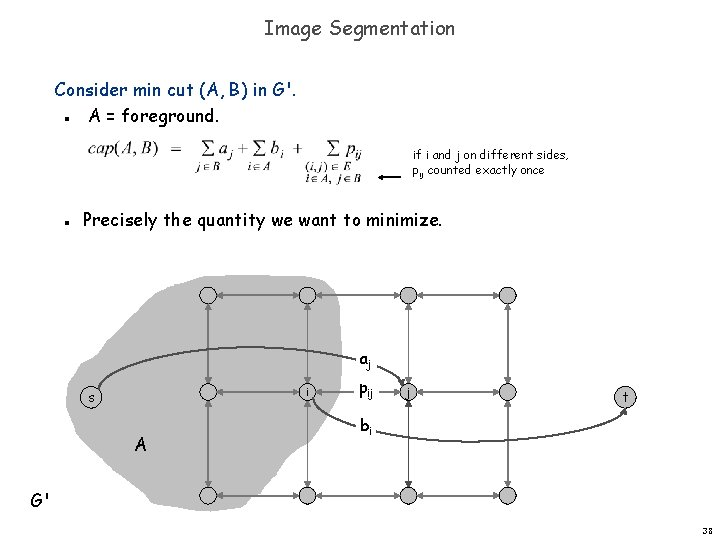Image Segmentation Consider min cut (A, B) in G'. A = foreground. n if i and j on different sides, pij counted exactly once n Precisely the quantity we want to minimize. aj i s A pij j t bi G' 38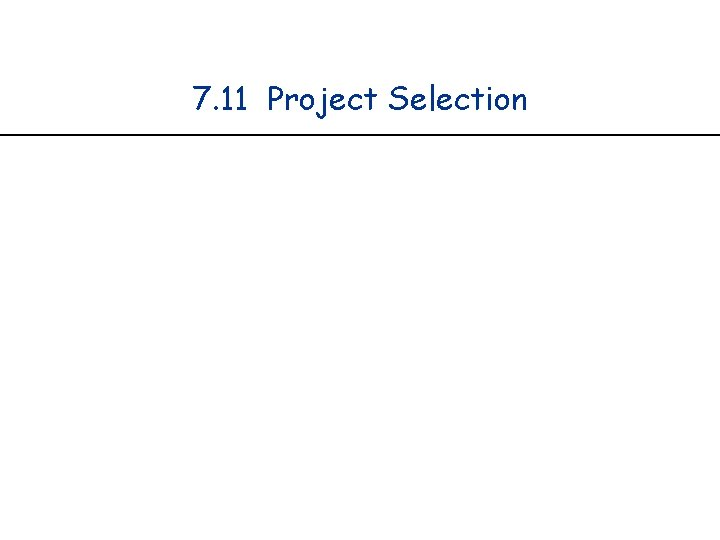7. 11 Project Selection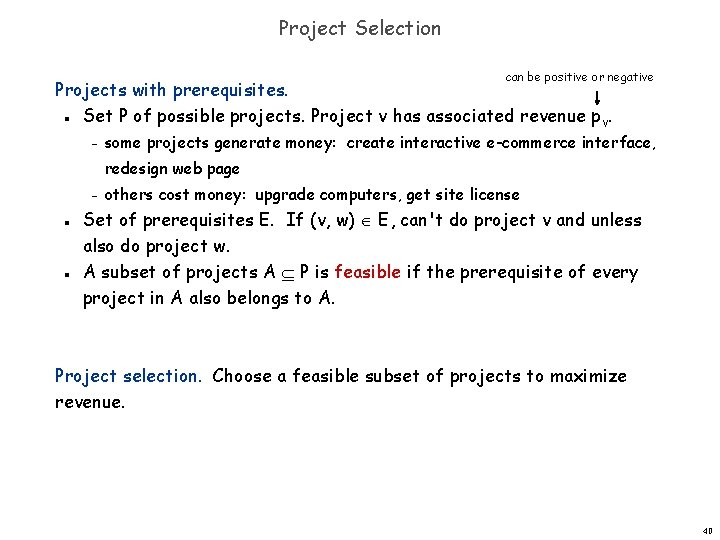Project Selection can be positive or negative Projects with prerequisites. Set P of possible projects. Project v has associated revenue p v. n – some projects generate money: create interactive e-commerce interface, redesign web page – n n others cost money: upgrade computers, get site license Set of prerequisites E. If (v, w) E, can't do project v and unless also do project w. A subset of projects A P is feasible if the prerequisite of every project in A also belongs to A. Project selection. Choose a feasible subset of projects to maximize revenue. 40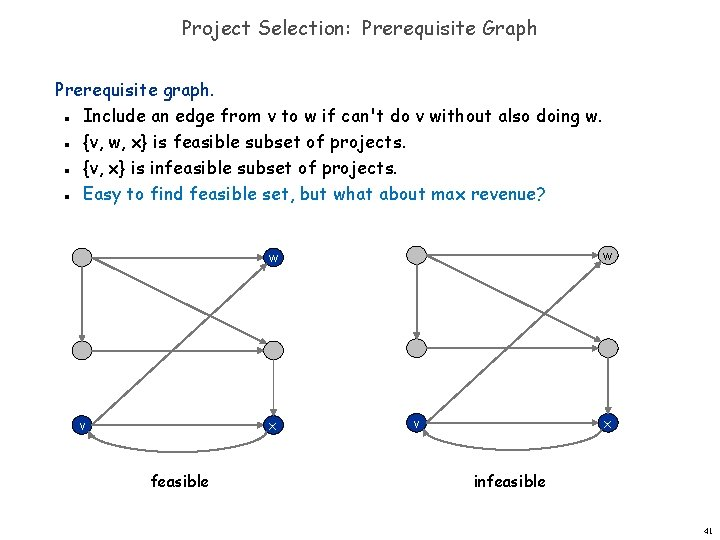Project Selection: Prerequisite Graph Prerequisite graph. Include an edge from v to w if can't do v without also doing w. {v, w, x} is feasible subset of projects. {v, x} is infeasible subset of projects. Easy to find feasible set, but what about max revenue? n n w w v x feasible v x infeasible 41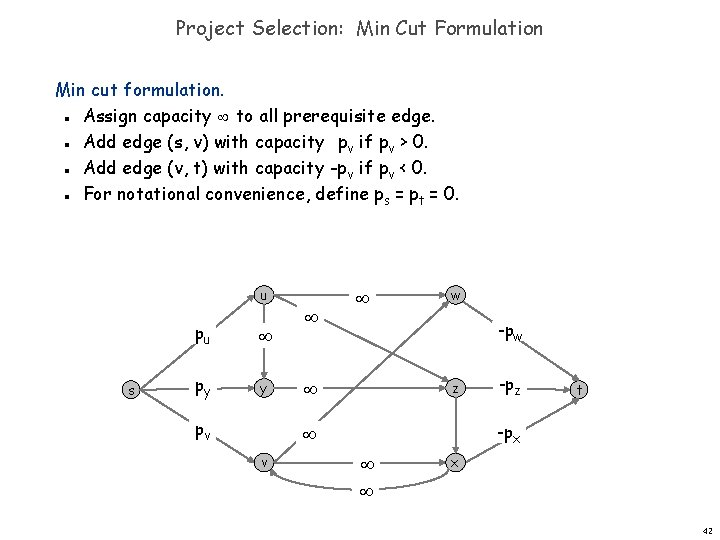Project Selection: Min Cut Formulation Min cut formulation. Assign capacity to all prerequisite edge. Add edge (s, v) with capacity -pv if pv > 0. Add edge (v, t) with capacity -pv if pv < 0. For notational convenience, define ps = pt = 0. n n u s pu py y pv w -pw z t -px v -pz x 42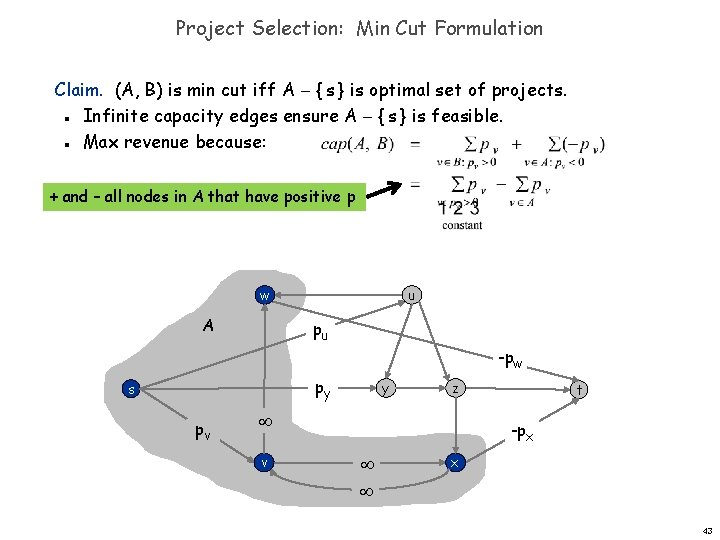Project Selection: Min Cut Formulation Claim. (A, B) is min cut iff A { s } is optimal set of projects. Infinite capacity edges ensure A { s } is feasible. Max revenue because: n n + and – all nodes in A that have positive p w A u pu -pw py s pv y z v t -px x 43Project selection application#1: Open Pit Mining Open-pit mining. (studied since early 1960 s) Blocks of earth are extracted from surface to retrieve ore. Each block v has net value pv = value of ore - processing cost. Can't remove block v before w or x. n n n w x v 447. 12 Baseball Elimination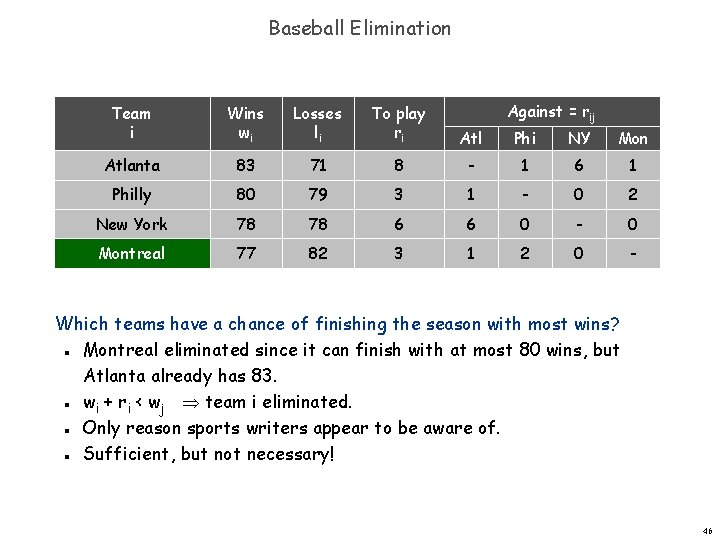Baseball Elimination Against = rij Team i Wins wi Losses li To play ri Atl Phi NY Mon Atlanta 83 71 8 - 1 6 1 Philly 80 79 3 1 - 0 2 New York 78 78 6 6 0 - 0 Montreal 77 82 3 1 2 0 - Which teams have a chance of finishing the season with most wins? Montreal eliminated since it can finish with at most 80 wins, but Atlanta already has 83. wi + ri < wj team i eliminated. Only reason sports writers appear to be aware of. Sufficient, but not necessary! n n 46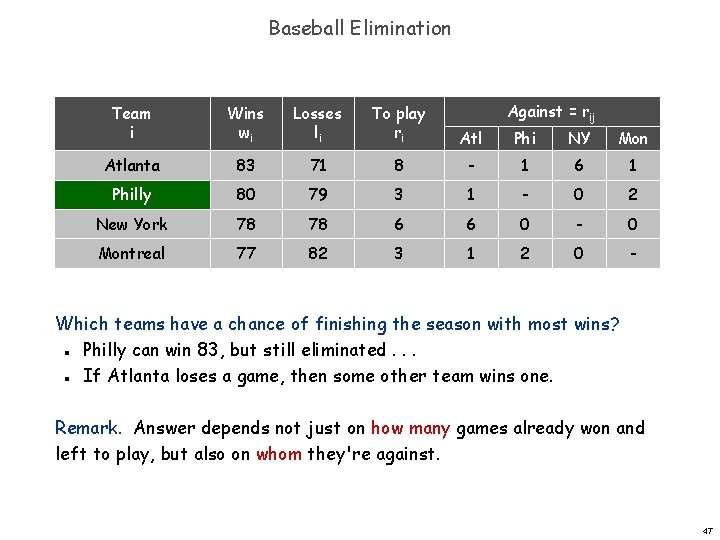Baseball Elimination Against = rij Team i Wins wi Losses li To play ri Atl Phi NY Mon Atlanta 83 71 8 - 1 6 1 Philly 80 79 3 1 - 0 2 New York 78 78 6 6 0 - 0 Montreal 77 82 3 1 2 0 - Which teams have a chance of finishing the season with most wins? Philly can win 83, but still eliminated. . . If Atlanta loses a game, then some other team wins one. n n Remark. Answer depends not just on how many games already won and left to play, but also on whom they're against. 47Baseball Elimination Baseball elimination problem. Set of teams S. Distinguished team s S. Team x has won wx games already. Teams x and y play each other rxy additional times. Is there any outcome of the remaining games in which team s finishes with the most (or tied for the most) wins? n n n 48Baseball Elimination: Max Flow Formulation Can team 3 finish with most wins? Assume team 3 wins all remaining games w 3 + r 3 wins. Find a solution of remaining games so that all teams have w 3 + r 3 wins. n n 1 -2 1 team 4 can still win this many more games 1 -4 2 games left 1 -5 s r 24 = 7 2 -4 4 w 3 + r 3 - w 4 t 2 -5 5 game nodes 4 -5 team nodes 49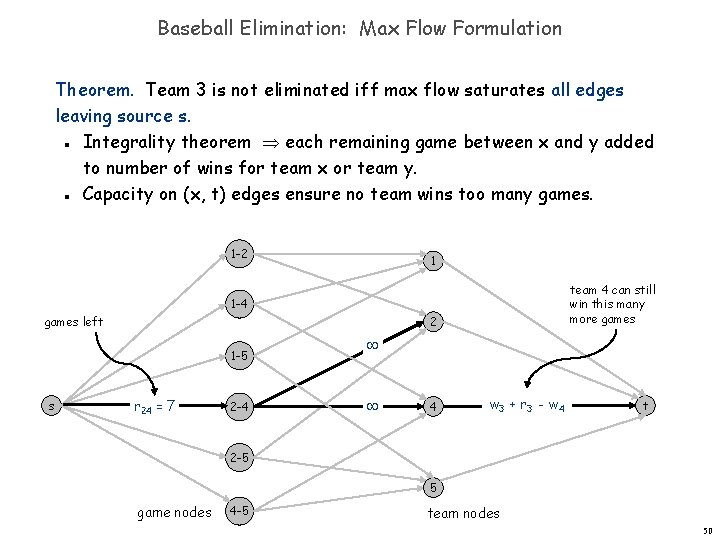Baseball Elimination: Max Flow Formulation Theorem. Team 3 is not eliminated iff max flow saturates all edges leaving source s. Integrality theorem each remaining game between x and y added to number of wins for team x or team y. Capacity on (x, t) edges ensure no team wins too many games. n n 1 -2 1 team 4 can still win this many more games 1 -4 2 games left 1 -5 s r 24 = 7 2 -4 4 w 3 + r 3 - w 4 t 2 -5 5 game nodes 4 -5 team nodes 50